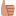Would you like to react to this message? Create an account in a few clicks or log in to continue.

# SimulIDE_0.4.15-SR10 ATTiny85 always reading zero volts

2 posters

#### eugenet

• Potentiometer connected to pin PB5.
• analogRead(PB5) always return a zero.
• Sample program below demonstrates the problem.

If analogRead is zero, It turns on LED on pin PB0. Otherwise the LED on pin PB1 is turned on instead.
Code:
`void setup() {  pinMode(PB5, INPUT);  DDRB |= (1 << PB0);  // Set pin PB0 for output  DDRB |= (1 << PB1);  // Set pin PB1 for output}void loop() {  if (analogRead(PB5)==0) {    PORTB |= (1 << PB0);  // PB0 LED ON    PORTB &= ~(1 << PB1); // PB1 LED OFF  } else {    PORTB &= ~(1 << PB0); // PB0 LED OFF    PORTB |= (1 << PB1);  // PB1 LED ON  }}`#### arcachofoHi.
I see a problem in your circuit:
10KOhm Potentiometer should be connected to ground, not to 100 Ohm resistor.

If this does not solve the problem let me know.

#### eugenet

Thanks, that fixed the problem.arcachofo likes this post